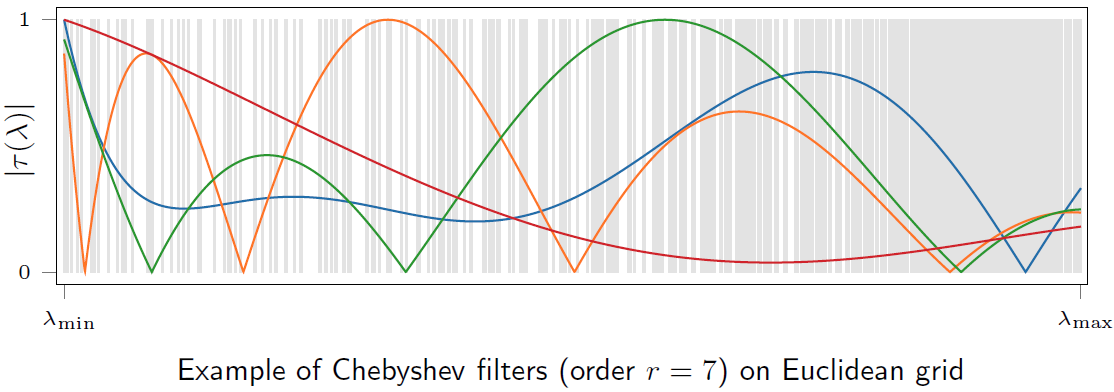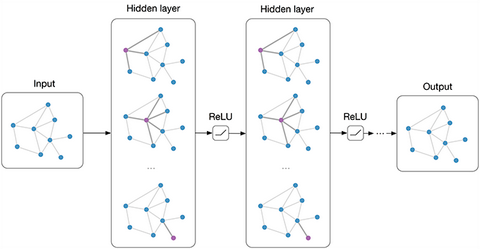• 由于（原因为拉普拉斯矩阵半正定，特征值非负），除以最大特征值，就转化在区间
• 再进行，就实现了目标。于是就有：（最大特征值可以利用幂迭代法（power iteration）求出，详细内容可以参考cnblogs.com/fahaizhong/• 当K=1时，卷积核为def chebyshev_polynomials(adj, k):
"""Calculate Chebyshev polynomials up to order k. Return a list of sparse matrices (tuple representation)."""
print("Calculating Chebyshev polynomials up to order {}...".format(k))

largest_eigval, _ = eigsh(laplacian, 1, which='LM') # \lambda_{max}
scaled_laplacian = (2. / largest_eigval) * laplacian - sp.eye(adj.shape) # 2/\lambda_{max}L-I_N

# 将切比雪夫多项式的 T_0(x) = 1和 T_1(x) = x 项加入到t_k中
t_k = list()
t_k.append(scaled_laplacian)

# 依据公式 T_n(x) = 2xT_n(x) - T_{n-1}(x) 构造递归程序，计算T_2 -> T_k项目
def chebyshev_recurrence(t_k_minus_one, t_k_minus_two, scaled_lap):
s_lap = sp.csr_matrix(scaled_lap, copy=True)
return 2 * s_lap.dot(t_k_minus_one) - t_k_minus_two

for i in range(2, k+1):
t_k.append(chebyshev_recurrence(t_k[-1], t_k[-2], scaled_laplacian))

return sparse_to_tuple(t_k)

superbrother：解读三种经典GCN中的Parameter Sharingzhuanlan.zhihu.comPlease wait whilst we redirect youauthors.elsevier.com

Zhang, Z., Li, M., Lin, X., & Wang, Y. (2020). Network-wide traffic flow estimation with insufficient volume detection and crowdsourcing data.Transportation Research Part C: Emerging Technologies,121, 102870.